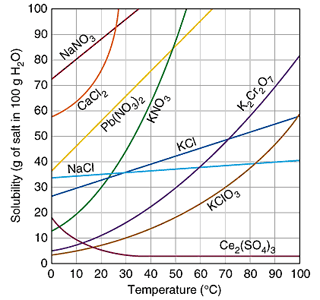sourceSolubility curves are graphs that show how much solute can dissolve in 100 g of water. Usually, many substances are shown on a single solubility curve. On the solubility curve above, nine different substances are shown.

On all solubility curves, temperature is on the x-axis, because it is the independent variable. It is the variable that we can control. The dependent variable goes on the y-axis. This is the variable that responds to the independent variable. Solubility is on the y-axis. Note that the solubility is measured as the grams of solute in 100 g of water.

Notice on the solubility curve that for most substances, the solubility increases as the temperature increases. For which substance is this not true?

Ce2(SO4)3

Solubility curves tell us at what point a solution is saturated, unsaturated, or supersaturated. Any point that falls on the line or curve for a solute would be a point of saturation. Any point that falls below the line or curve for a solute would represent an unsaturated solution. Any point that falls above the line or curve for a solute would represent a supersaturated solution.

For the questions below, an amount of solute is given and the temperature is stated. If all of the solute could be dissolved in 100 g of water at the given temperature, would the resulting solution be unsaturated, saturated, or supersaturated? Use the solubility curve:source1. 60 g KCL at 70 °C

supersaturated

2. 10 g KCLO3 at 60 °C

unsaturated

3. 80 g NaNO3 at 10 °C

saturated

4. 70 g CaCl2 at 20 °C

unsaturated

For the questions below, a solute and temperature are given. Tell approximately how many grams of each solute must be added to 100 g of water to form a saturated solution at the given temperature.

5. Pb(NO3)2 at 10 °C

~ 46 g

6. Ce2(SO4)3 at 50 °C

~3 g

7. NaCl at 20 °C

~35 g

8. K2Cr2O7 at 50 °C

~30 g

Which solution is more concentrated?

9. At 10 °C: a saturated solution of KNO3 or a saturated solution of CaCl2.

a saturated solution of CaCl2

10. At 50 °C: a saturated solution of KNO3 or an unsaturated solution of NaNO3 consisting of 90 g of the solute dissolved in 100 g of water.

Answer</summaryan unsaturated solution of NaNO3 consisting of 90 g of the solute dissolved in 100 g of water

11. If 115 g KNO3 are added to 100 g of water at 35 °C, how many grams do not dissolve?

115 g are added. From the graph, ~54 g of KNO3 will dissolve in 100 g of water at 35 degree C

12. What mass of KCl would be needed to form a saturated solution if the KCl was dissolved in 200 g of water at 80 °C?